# Applications of Calculus: Derivatives in String Art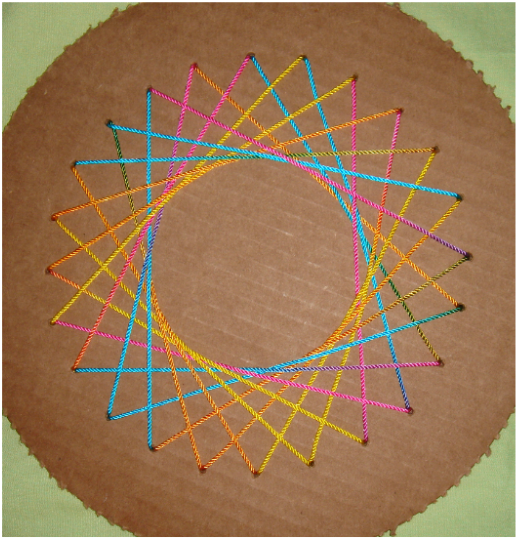String art involves creating curves, using only straight lines. The reason it works is because the lines “slope” into each other, becoming tangent lines to the curve. For example, the lines above are the tangent lines of the circle, where the slope of the line matches the derivative of the circle at each point.

Next, tangent lines to an exponential function: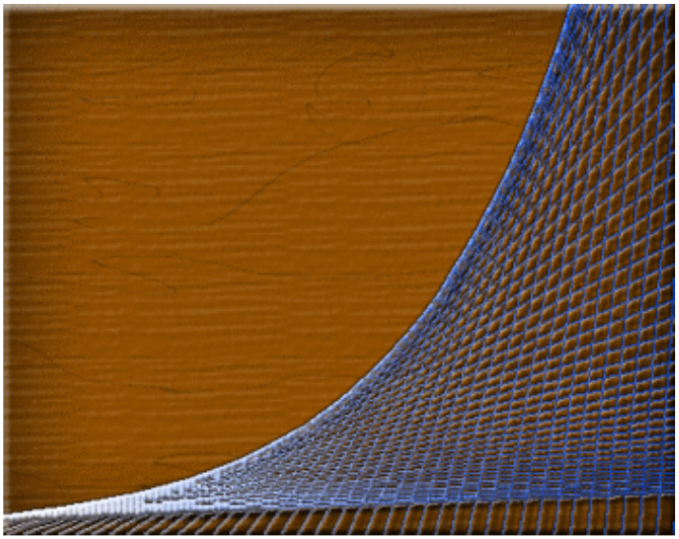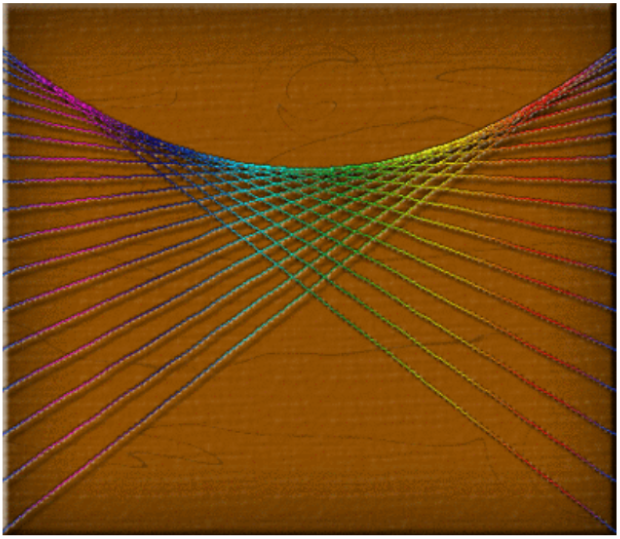A cubic: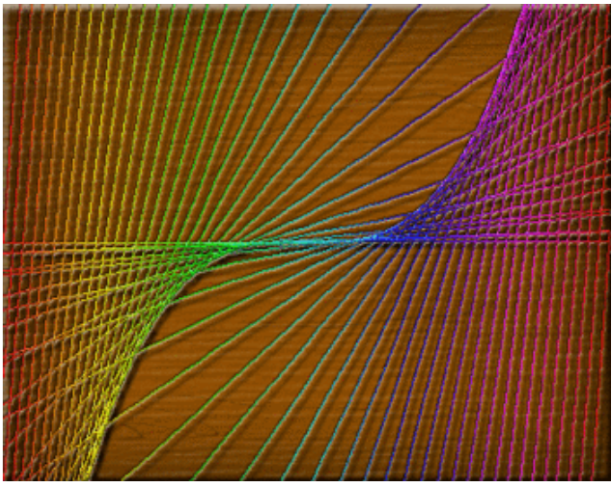And finally, a sine wave: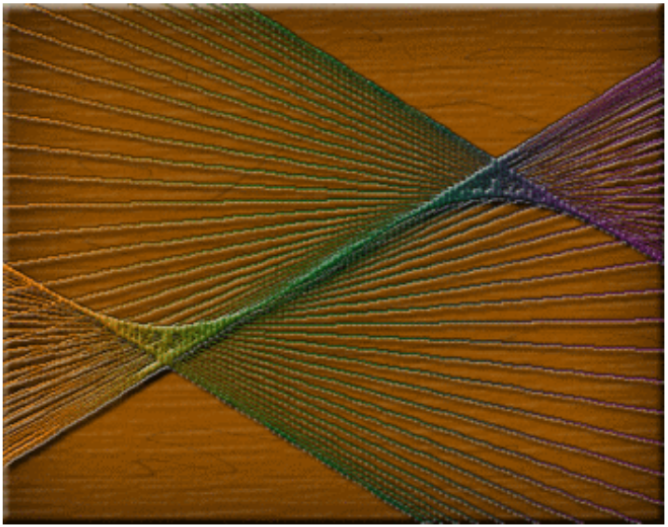Image credits: Math Images - The Math Forum @ Drexel
http://mathforum.org/mathimages/index.php/String_Art_Calculus

Tags: